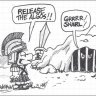# Short Swing Trade Indicator for ThinkorSwim##### Well-known member
I'm no coder myself but I still like to make strategies out of other indicators. Here is a simple one I whipped up today using the TMO and MACD. Its goal is to catch signals slightly before the macd and play off oversold/overbought zones on the TMO.

The OB/OS values make this indicator, set lower values and the criteria is less strict and therefore sometimes produces less consistent signals. Put it closer to 1 and it will make signals rarer yet more effective. Right now I have the settings at .9 for overbought and .6 for oversold. These are uneven because I believe it better fits the current bull market. If you are using this on a stock, understand its long term trend and adjust the zones if you wish. For example on a stock like GME crank the OS value up to something like .9, then make the OB value something like .6. If these zones are adequately adjusted I'd say it has around 60% accurate signals.

I haven't had much time to test the indicator but it looks solid on the 1d. I plan on using this to scan for stocks that could be promising. Along with some basing trading knowledge I think the signal accuracy could be boosted to around 80%. This is very simple and I'm sure there are many other ways it can be improved. Do you guys have any ideas? Also I was thinking wondering if I could draw any connections between this and the market moves indicator, does anyone have a version that scales properly? When I put an sma over a rsi is doesn't work.The code is not organized at all, nor are the inputs. I'm not good at sorting through that kind of thing. Bullish and bearish are the only plots that should be used.
Code:
``````def c = close;
def h = high;
def l = low;
def o = open;

input length = 14;
input calcLength = 5;
input smoothLength = 3;

def op = open;
def cl = close;
def data = fold i = 0 to length
with s
do s + (if cl > GetValue(op, i)
then 1
else if cl < GetValue(op, i)
then - 1
else 0);
def EMA5 = ExpAverage(data, calcLength);
plot Main = ExpAverage(EMA5, smoothLength);
plot Signal = ExpAverage(Main, smoothLength);
Main.AssignValueColor(if Main > Signal
then Color.GREEN
else Color.RED);
Signal.AssignValueColor(if Main > Signal
then Color.GREEN
else Color.RED);

def zero = if IsNaN(cl) then Double.NaN else 0;

def obtmo = if IsNaN(cl) then Double.NaN else Round(length * .85);

def ostmo = if IsNaN(cl) then Double.NaN else -Round(length * .60);

input fastLength = 12;
input slowLength = 26;
input MACDLength = 9;
input averageType2 = averageType.Exponential;
input showBreakoutSignals = no;

def Value = MovingAverage(averageType2, close, fastLength) - MovingAverage(averageType2, close, slowLength);
def Avg = MovingAverage(averageType2, Value, MACDLength);

def Diff = Value - Avg;
def ZeroLine = 0;

def Condition1 = if
Value  < Avg  and
Value > Avg
then 1 else Double.NaN;
plot x = Condition1;
x.SetPaintingStrategy(paintingStrategy = PaintingStrategy.BOOLEAN_ARROW_UP);

def Condition2 = if
Value  > Avg  and
Value < Avg
then 1 else Double.NaN;
plot q = Condition2;
q.SetPaintingStrategy(paintingStrategy = PaintingStrategy.BOOLEAN_ARROW_DOWN);

#
# TD Ameritrade IP Company, Inc. (c) 2007-2019
#
input lengthrsi = 14;
input over_Bought = 70;
input over_Sold = 30;
input price = close;
input averageType = averageType.Wilders;
input showBreakoutSignalsrsi = no;

def NetChgAvg = MovingAverage(averageType, price - price, length);
def TotChgAvg = MovingAverage(averageType, AbsValue(price - price), length);
def ChgRatio = if TotChgAvg != 0 then NetChgAvg / TotChgAvg else 0;
plot RSI = 50 * (ChgRatio + 1);
plot OverSold = over_Sold;
plot OverBought = over_Bought;
plot UpSignal = if RSI crosses above OverSold then OverSold else Double.NaN;
plot DownSignal = if RSI crosses below OverBought then OverBought else Double.NaN;

UpSignal.SetHiding(!showBreakoutSignals);
DownSignal.SetHiding(!showBreakoutSignals);

RSI.DefineColor("OverBought", GetColor(5));
RSI.DefineColor("Normal", GetColor(7));
RSI.DefineColor("OverSold", GetColor(1));
RSI.AssignValueColor(if RSI > over_Bought then RSI.Color("OverBought") else if RSI < over_Sold then RSI.Color("OverSold") else RSI.Color("Normal"));
OverSold.SetDefaultColor(GetColor(8));
OverBought.SetDefaultColor(GetColor(8));
UpSignal.SetDefaultColor(Color.UPTICK);
UpSignal.SetPaintingStrategy(PaintingStrategy.ARROW_UP);
DownSignal.SetDefaultColor(Color.DOWNTICK);
DownSignal.SetPaintingStrategy(PaintingStrategy.ARROW_DOWN);

plot bullish = (Main > Signal) and (Signal < ostmo);
plot bearish = (Main < Signal) and (Signal > obtmo);

#STRATEGY
#def SS = if bullish then 100 else if bearish then -100 else 0

# def sBuy = SS crosses above 0;;
# def sSell = SS crosses below 0;

# AddOrder(OrderType.BUY_to_OPEN, condition = bullish, price = open[-1], 100, tickcolor = Color.GREEN, arrowcolor = Color.GREEN);
# AddOrder(Ordertype.SELL_TO_CLOSE, condition = bearish2, price = open[-1], 100, tickcolor = Color.GREEN,
# arrowcolor = Color.GREEN);
#AddOrder(OrderType.SELL_AUTO, condition = bearish, price = open[-1], 1000, tickcolor = Color.RED, arrowcolor = Color.RED);
#END OF trend, a momentum and a cycle based indicator for ThinkorSwim``````

•markos, BenTen and giorgio##### Well-known member
I'll be posting a swing trade watchlist when I can.

•giorgio#### horserider

##### Well-known member
VIP
@YungTraderFromMontana Use the Ultimate RSI for your RSI and SMA combo. Just use the RSI plot and midline plot. I think there is a slimmed down version posted on that thread which will do what you want. Yes, cannot combine RSI and an SMA without having scaling issues.

•deleted

Last edited:
A

#### alphabeta

##### Member
is there any best indicators fpr 1 m chart#### markos

##### Well-known member
VIP
is there any best indicators fpr 1 m chart
Hi & welcome. This question should be placed in the questions area so that it is seen as a new thread.

•alphabeta